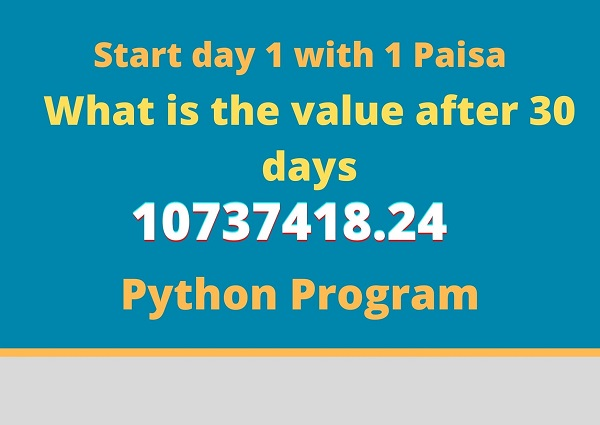# Python Program | How much money would you make if you would start with .01P double that number every day

Last updated Jan 03, 2021

Do you think How much money would you make when we start money with 1 Paisa and double the value to the next day.

With a small python program, we will calculate the amount# Python program to net amount after 30 days money=.01 for x in range(0,30):     money = money*2;     print("day % s = % s" % (x+1, money)) print("========== ==========")   print("Total = % s"%(money))

In this python program, we were used for loop with range function. Concatenate String with Number by '%' operator.

Output:

 day 1 = 0.02 day 2 = 0.04 day 3 = 0.08 day 4 = 0.16 day 5 = 0.32 day 6 = 0.64 day 7 = 1.28 day 8 = 2.56 day 9 = 5.12 day 10 = 10.24 day 11 = 20.48 day 12 = 40.96 day 13 = 81.92 day 14 = 163.84 day 15 = 327.68 day 16 = 655.36 day 17 = 1310.72 day 18 = 2621.44 day 19 = 5242.88 day 20 = 10485.76 day 21 = 20971.52 day 22 = 41943.04 day 23 = 83886.08 day 24 = 167772.16 day 25 = 335544.32 day 26 = 671088.64 day 27 = 1342177.28 day 28 = 2684354.56 day 29 = 5368709.12 day 30 = 10737418.24 ========== ========== Total = 10737418.24

Oh, know What is the price after 30 days when we start at 1 paisa, It's Exactly 10737418.24 Rs

What is the Big Number we will get If we start 2021 with 1Rs and After the End of 2021

 money=1 for x in range(0,365):     money = money*2;     print("day % s = % s" % (x+1, money)) print("========== ==========")   print("Total = % s"%(money))

Is very Big number  75153362648762663292463379097258784876021841565066235862633311089030688803667470190838367948312598497021919232

But it's not possible in Real life to add double to the Next day.

 Article Contributed By :509 Views